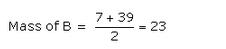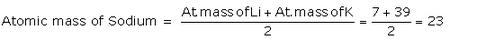Courses

# Lakhmir Singh & Manjit Kaur: Periodic Classification Of Elements, Solutions- 1 Class 10 Notes | EduRev

## Class 10 : Lakhmir Singh & Manjit Kaur: Periodic Classification Of Elements, Solutions- 1 Class 10 Notes | EduRev

The document Lakhmir Singh & Manjit Kaur: Periodic Classification Of Elements, Solutions- 1 Class 10 Notes | EduRev is a part of the Class 10 Course Class 10 Chemistry Solutions By Lakhmir Singh & Manjit Kaur.
All you need of Class 10 at this link: Class 10

(Page No:281)

Question 1:
(a) On what basis did Mendeleev arrange the elements in his periodic table ?
(B) On what basis are they arranged now ?
Solution :

(a) Mendeleev arrange the element in his periodic table on the basis of atomic masses.
(b) Now they are arranged on the basis of atomic numbers.

Question 2:
State whether the following statements are true or false :
(a) Newlands divided the elements into horizontal rows of eight elements each.
(b) According to Mendeleev’s periodic law, the properties of elements are a periodic function of their atomic numbers.
(c) The elements in a group have consecutive atomic numbers.
Solution :

(a) False
(b) False
(c) False

(Page No:282)

Question 3:
Name the Russian chemist who said that the properties of elements are a periodic function of their atomic masses.
Solution :

Mendeleev said that the properties of elements are a periodic function of their atomic masses.

Question 4:
Rewrite the following statements after correction, if necessary :
(a) Groups have elements with consecutive atomic numbers.
(b) Periods are the horizontal rows of elements.
(c) Isotopes are the elements of the same group.
Solution :

(a) Periods have elements with consecutive atomic numbers.
(b) Correct.
(c) Correct.

Question 5:
Name the scientists who gave the following laws in the early classification of elements :
Law of octaves (b) Law of triads
Solution :

(a) Newlands.
(b) Dobereiner.

Question 6:
A, B and C are the elements of a Dobereiner’s triad. If the atomic mass of A is 7 and that of C is 39, what should be the atomic mass of B ?
Solution :Question 7:
X and Y are the two elements having similar properties which obey Newlands’ law of octaves. How many elements are there in-between X and Y ?
Solution :

Six elements.

Question 8:
What was Mendeleev’s basis for the classification of elements ?
Solution :

Mendeleev’s basis for the classification of elements was the atomic mass of elements.

Question 9:
In the classification of the then-known elements, Mendeleev was guided by two factors. What are those two factors?
Solution :

Mendeleev was guided by two factors:
(i) Increasing atomic masses.
(ii) Grouping together of elements having similar properties.

Question 10:
Name two elements whose properties were predicted on the basis of their positions in Mendeleev’s periodic table.
Solution :

Gallium and Scandium.

Question 11:
The three elements predicted by Mendeleev from the gaps in his periodic table were known as eka-boron, eka-aluminium and eka-silicon. What names were given to these elements when they were discovered later on ?
Solution :

Scandium, Gallium, Germanium.

Question 12:
Name two elements whose properties were predicted on the basis of their positions in Mendeleev’s periodic table.
Solution :

Eka-Aluminium (gallium) and Eka-Silicon (germanium).

Question 13:
State one example of a Dobereiner’s triad, showing in it that the atomic mass of a middle element is halfway between those of the other two.
Solution :

The elements lithium, sodium and potassium form a Dobereiner’s triad. Lithium is the first element of this triad, sodium is the middle element whereas potassium is the third element of the triad. Sodium (middle element) has atomic mass 23.
According to Dobereiner,Question 14:
Which group of elements could be placed in Mendeleev’s periodic table later on, without disturbing the original order ? Give reason.
Solution :

Noble gases. Since they are chemically unreactive, so they got a place in the periodic table in the form of a separate group and hence did not disturb the original order of Mendeleev’s periodic table

Question 15:
Fill in the following blanks with suitable words :
(a) The basis for modern periodic table is………
(b) The horizontal rows in a periodic table are called……….
(c) Group 1 elements are called………
(d) Group 17 elements are known as………
(e) Group 18 elements are called…….
(f) According to Newlands’ classification of elements, the properties of sulphur are similar to those of oxygen
because sulphur is the………. element starting from oxygen.
Solution :

(a) Atomic number .
(b) Periods.
(c) Alkali metals .
(d) Halogens .
(e) Noble Gases .
(f) Eighth.

Question 16:
(a) What is meant by (i) a group, and (ii) a period, in a periodic table ?
(b) How many periods and groups are there in the long form of periodic table ?
(c) Give two examples each of (i) group 1 elements (ii) group 17 elements (iii) group 18 elements.
Solution :

(a)(i) The vertical columns in a periodic table are called groups.
(ii) The horizontal rows of elements in a periodic table are called periods.
(b) There are seven periods and eighteen groups in the long form of periodic table.
(c)(i) Group 1: Lithium and Sodium.
(ii) Group 17: Fluorine and Chlorine.
(iii) Group 18: Neon and Argon.

Question 17:
(a) In the modern periodic table, which are the metals among the first ten elements ?
(b) What is the significance of atomic number in the modern classification of elements ? Explain with the help of an example.
Solution :

(a) Lithium, Beryllium are metals.
(b) The real significance of atomic number in the modern periodic classification is that it relates the periodicity in the properties of elements to the periodicity in their electronic configurations.
Example: The atomic number increases from 3 in lithium to 11 in sodium, there is a repetition of electronic configuration from 2,1 to 2,8,1 (both having 1 valence electron).

Question 18:
(a) How were the positions of isotopes of an element decided in the modern periodic table ?
(b) How were the positions of cobalt and nickel resolved in the modern periodic table ?
(c) Where should hydrogen be placed in the modern periodic table ? Give reason for your answer.
Solution :

(a) Position of isotopes: All the isotopes of an element have the same number of protons, so their atomic number is also the same. Since, all the isotopes of an element have the same atomic number; they can be put at one place in the same group of the periodic table.
(b) Position of Cobalt and Nickel: The atomic number of cobalt is 27 and that of nickel is 28. According to modern periodic law, the elements are arranged in order of increasing atomic numbers. So, cobalt with lower atomic number (27) should come first and nickel with higher atomic number (28) should come later, even if their atomic masses are in the wrong order.
(c) Position of hydrogen: Hydrogen has been placed at the top of group 1, above the alkali metals in the modern periodic table because the electronic configuration of hydrogen is similar to those of alkali metals. Both, have 1 valence electron each.

Question 19:
(a) On which side of the periodic table will you find metals ?
(b) On which side of the periodic table will you find non-metals ?
(c) What is the name of those elements which divide metals and non-metals in the periodic table ?
Solution :

(a) Left side.
(b) Right side.
(c) Metalloids.

Offer running on EduRev: Apply code STAYHOME200 to get INR 200 off on our premium plan EduRev Infinity!

20 docs

,

,

,

,

,

,

,

,

,

,

,

,

,

,

,

,

,

,

,

,

,

,

,

,

;